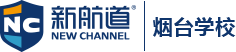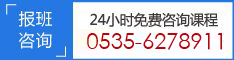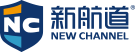15年专注雅思、托福、SAT等出国语言考试培训### 物理常用术语词汇

A

Absolute zero

The lowest theoretical temperature a material can have, where the molecules that make up the material have no kinetic energy. Absolute zero is reached at 0 K or –273o C.

Acceleration

A vector quantity defined as the rate of change of the velocity vector with time.

Activity

In radioactive substances, the number of nuclei that decay per second. Activity, A, will be larger in large samples of radioactive material, since there will be more nuclei.

Alpha decay

A form of radioactive decay where a heavy element emits an alpha particle and some energy, thus transforming into a lighter, more stable, element.

Alpha particle

A particle, which consists of two protons and two neutrons. It is identical to the nucleus of a helium atom and is ejected by heavy particles undergoing alpha decay.

Amplitude

In reference to oscillation, amplitude is the maximum displacement of the oscillator from its equilibrium position. Amplitude tells how far an oscillator is swinging back and forth. In periodic motion, amplitude is the maximum displacement in each cycle of a system in periodic motion. The precise definition of amplitude depends on the particular situation: in the case of a stretched string it would be measured in meters, whereas for sound waves it would be measured in units of pressure.

Angle of incidence

When a light ray strikes a surface, the angle of incidence is the angle between the incident ray and the normal.

Angle of reflection

The angle between a reflected ray and the normal.

Angle of refraction

The angle between a refracted ray and the line normal to the surface.

Angular acceleration

A vector quantity, , equal to the rate of change of the angular velocity vector with time. It is typically given in units of rad/s2.

Angular displacement

The net change, , in a point’s angular position, . It is a scalar quantity.

Angular frequency

A frequency, f, defined as the number of revolutions a rigid body makes in a given time interval. It is a scalar quantity commonly denoted in units of Hertz (Hz) or s–1.

Angular momentum

A vector quantity, L, that is the rotational analogue of linear momentum. For a single particle, the angular momentum is the cross product of the particle’s displacement from the axis of rotation and the particle’s linear momentum, . For a rigid body, the angular momentum is a product of the object’s moment of inertia, I, and its angular velocity, .

Angular period

The time, T, required for a rigid body to complete one revolution.

Angular position

The position of an object according to a co-ordinate system measured in s of the angle of the object from a certain origin axis. Conventionally, this origin axis is the positive x-axis.

Angular velocity

A vector quantity, , that reflects the change of angular displacement with time, and is typically given in units of rad/s. To find the direction of the angular velocity vector, take your right hand and curl your fingers along the particle or body’s direction of rotation. Your thumb then points in the direction of the body’s angular velocity.

Antinode

The points midway between nodes on a standing wave, where the oscillations are largest.

Atom

The building blocks of all matter, atoms are made up of a nucleus consisting of protons and neutrons, and a number of electrons that orbit the nucleus. An electrically neutral atom has as many protons as it has electrons.

Atomic number

A number, Z, associated with the number of protons in the nucleus of an atom. Every element can be defined in s of its atomic number, since every atom of a given element has the same number of protons.

Axis of rotation

The line that every particle in the rotating rigid body circles about.

### 推荐阅读

【本文标签】：

【责任编辑】：青岛新航道版权所有：转载请注明出处

### SAT考友经验### 客服热线 0535-6278911